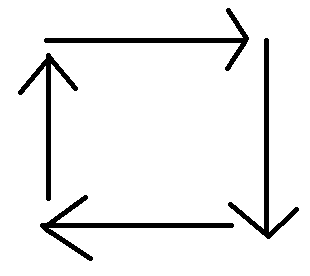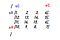# CoDEVIANT #21 (10/11/20) — Spiral Motion

So I’ve got a neat doozy of a problem to write about here.

We are to write a problem that takes a two-dimensional array and returns an array of the values when the values are read in a spiral motion.You spin me right round, baby, right round like algorithm, baby right right round… XD

So…I got a confession to make. I couldn’t figure out how to solve it by myself. After a number of failed attempts, I swallowed my pride and consulted the experts. But I learned a lot and I’m excited to share some knowledge with you!

Before I share the answer, I want to give an example of the kind of information we’ll be providing as an argument to the function.

Assuming we pass in:

`[   [1,   2,  3, 4],   [12, 13, 14, 5],   [11, 16, 15, 6],   [10,  9,  8, 7]]`

The function should push values to a new array like so

[1, 2, 3, 4, 5, 6, 7, 8, 9, 10, 11, 12, 13, 14, 15, 16]

Notice that we avoid repeating any of the values. We achieve this by the following steps:

• Read the top row from left to right, including the far right value.
• Read the far right column omitting the very top value and including the very bottom value.
• Read the bottom row from right to left omitting the right-most value and including the left-most value.
• Rad the far left column, omitting the far left bottom value and the far left top value.

What we’re going to do is read the perimeter like this, then once we finish the outer perimeter, we (if there is one present) move into the inner perimeter.

Here’s the solution:

`function spiralTraverse(array) {  // Write your code here.  let results = [] let startCol = 0 let startRow = 0 //endRow signifies the start places of each row up and down the verticle side of the block let endRow = array.length - 1 //endCol signifies the start places of each column across the top of the block let endCol = array.length - 1  while(startCol <= endCol && startRow <= endRow) {  //top  for(let col = startCol; col <= endCol ; col++) {   results.push(array[startRow][col])   console.log('top', results)  }    //right  for(let row = startRow + 1; row <= endRow; row++) {   results.push(array[row][endCol])   console.log('right', results)  }    //bottom  for(let column = endCol - 1; column >= startCol; column --) {   if(startRow === endRow) break   results.push(array[endRow][column])   console.log('bottom', results)  }    //left  for(let row = endRow - 1; row > startRow; row --) {   if(startCol === endCol) break   results.push(array[row][startCol])   console.log(results)  }    startCol++  startRow++  endCol--  endRow-- } return results}`

First we create an empty array called results. Then we create some pointers signifying the coordinates that we’re going to use to point to the value in the two-dimensional array that we want to push into results.

`let results = [] let startCol = 0 let startRow = 0 //endRow signifies the start places of each row up and down the verticle side of the block let endRow = array.length - 1 //endCol signifies the start places of each column across the top of the block let endCol = array.length - 1`startColumn (sC) is 0 to start. startRow (sR) is 0 to start. endColumn (eC) is the length of the first array in the 2D array “1,2,3,4” minus one -3-. endRow (eR) is the length of the 2D array “1,12,11,10”. The value is 3 as well.

We are going to create a while-loop that will be active as long as startCol is less than OR equal to endCol AND startRow is less than OR equal to endRow. At the end of each iteration of this while loop, startCol and startRow will be incremented by one. endCol and endRow will be decremented by one. This prevents the while-loop from running infinitely.

`while(startCol <= endCol && startRow <= endRow) {   ...   startCol++   startRow++   endCol--   endRow--}`

Next we are going to set up for-loops that will read the values from the perimeter in the directions discussed above (lengths too). They are:

• top // left to right
• right //top to bottom
• bottom // left to right
• left //bottom to top

top

`for(let col = startCol; col <= endCol; col++) {   results.push(array[startRow][col])  }`

We say that col equals whatever startCol is at that moment. We keep the for-loop going as long as col is less-than or equal-to endCol at that moment. After each loop, col increments by one. Then we push whatever value is at
array[startRow], [col].
It would be like
array //(1),
array // (2),
array // (3),
array // (4)

right

`for(let row = startRow + 1; row <= endRow; row++) {   results.push(array[row][endCol])}`

We say that row equals startRow plus 1. This for-loop stays active so long as row is less-than or equal-to endRow. After each iteration, row increments by one. We then push the value of whatever array[row][endCol] is into results.
array // (5)
array // (6)
array // (7)

bottom

`for(let column = endCol - 1; column >= startCol; column --) {   if(startRow === endRow) break   results.push(array[endRow][column])   console.log('bottom', results)  }`

We say that column equals endCol minus one. The for-loop stays active as long as column is larger than or equal to startCol. After each iteration, column decrements by one. To avoid repeated integers, we say that if startRow equals endRow, then we break the loop. Meaning we jump out of it and move along with what’s going on in the parent while-loop. Anyways, in most cases we push whatever value we get from array[endRow][column].
At the start the values we’ll be pushing are:
array // (8)
array // (9)
array // (10)

left

`for(let row = endRow - 1; row > startRow; row --) {   if(startCol === endCol) break   results.push(array[row][startCol])   console.log(results)  }`

We then say that row equals endRow minus 1. The for-loop keeps running as long as row is larger than startRow. Each iteration has row decrementing by one. If startCol and endCol equal eachother, then the for-loop breaks. In most other cases, we push the value at array[row][startCol] into results:
array // (11)
array // (12)

At this point, the startRow and startColumn values increase by one. Then the endRow and endColumn values decrease by one. This makes it so that we can establish a new inner-perimeter so we can push the inner values into our results array.

And that’s basically how this problems works. It’s a pretty cool one and, on the outset, seems like a really impressive problem to be able to solve.

Stay frosty…

is a web developer, opera singer, actor, and lover of cats. (adrian-rosales.tech)